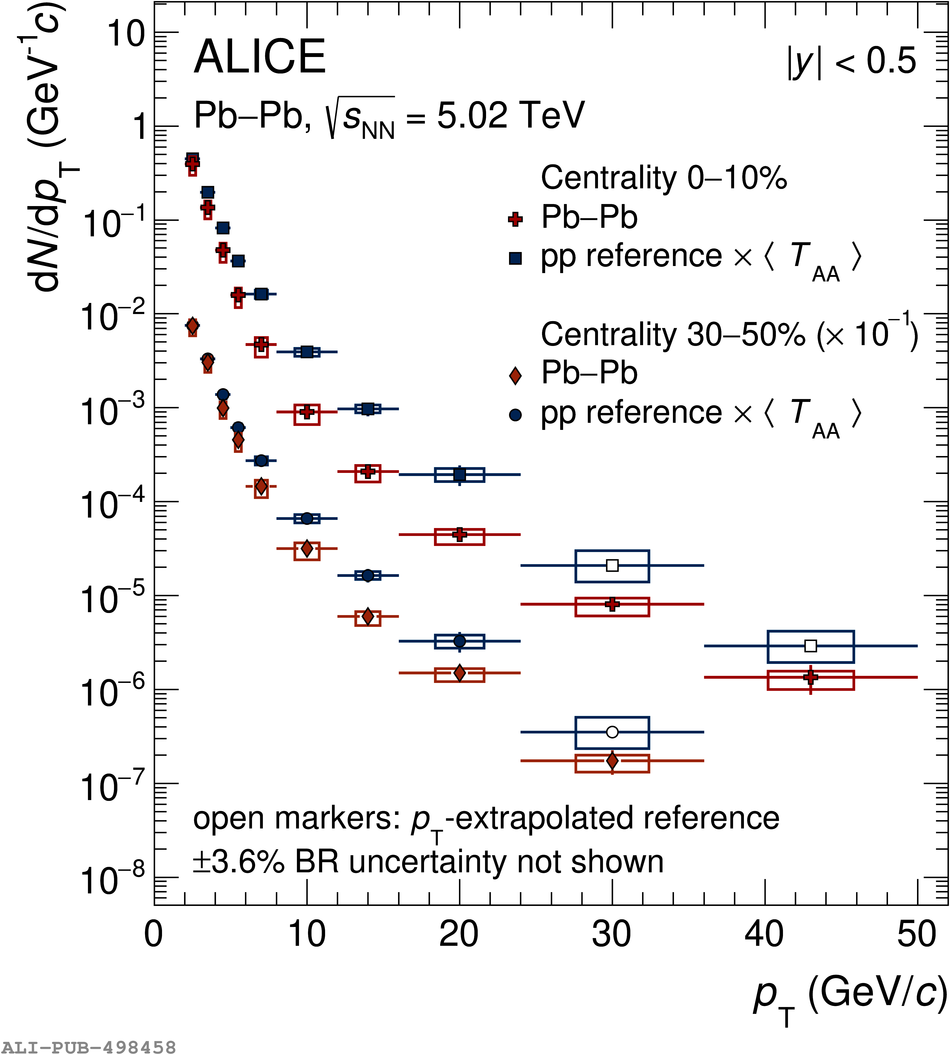Figure 3

 $\pt$-differential production yields of prompt $\Ds$ mesons in the 0--10\% and 30--50\% centrality intervals in Pb--Pb collisions at $\sqrtsNN=5.02~\TeV$ compared to the pp reference~ scaled by the average nuclear overlap function $\av{\TAA}$ of the corresponding centrality interval. The open markers indicate where the pp reference is extrapolated using FONLL calculations. The $\pt$-differential yields in the 30--50\% centrality interval and the corresponding pp reference are scaled by a factor of $10^{-1}$ for better visibility. Statistical uncertainties (bars) and systematic uncertainties (boxes) are shown.